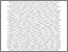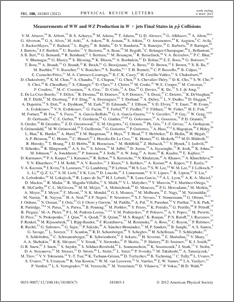# Measurements of WW and WZ production in W + jets final states in p-pbar collisions

Collaboration, D0 and Bertram, Iain and Borissov, Guennadi and Fox, Harald and Ross, Anthony and Williams, Mark and Ratoff, Peter (2012) Measurements of WW and WZ production in W + jets final states in p-pbar collisions. Physical review letters, 108 (18). ISSN 1079-7114Preview
PDF
PhysRevLett.108.181803.pdf - Published Version

## Abstract

We study WW and WZ production with $l\nu q{q}$ ($l=e,\mu$) final states using data collected by the D0 detector at the Fermilab Tevatron Collider corresponding to 4.3 fb^-1 of integrated luminosity from p-pbar collisions at sqrt{s}=1.96 TeV. Assuming the ratio between the production cross sections $\sigma(WW)$ and $\sigma(WZ)$ as predicted by the standard model, we measure the total WV (V=W,Z) cross section to be $\sigma(WV)= 19.6^{+3.2}_{-3.0}$ pb, and reject the background-only hypothesis at a level of 7.9 standard deviations. We also use b-jet discrimination to separate the WZ component from the dominant WW component. Simultaneously fitting WW and WZ contributions, we measure $\sigma(WW) = 15.9^{+3.7}_{-3.2}$ pb and $\sigma(WZ) = 3.3^{+4.1}_{-3.3}$ pb, which is consistent with the standard model predictions.

Item Type:
Journal Article
Journal or Publication Title:
Physical review letters
© 2012 American Physical Society 13 pages, 8 Figures, 3 Tables; To be submitted to Phys. Rev. Lett
Uncontrolled Keywords:
/dk/atira/pure/subjectarea/asjc/3100
Subjects:
Departments:
ID Code:
63561
Deposited By:
Deposited On:
24 Apr 2013 08:05
Refereed?:
Yes
Published?:
Published